Question

# Consider the block diagram as shown in Figure Q4(a) for the system under consideration. Assume the...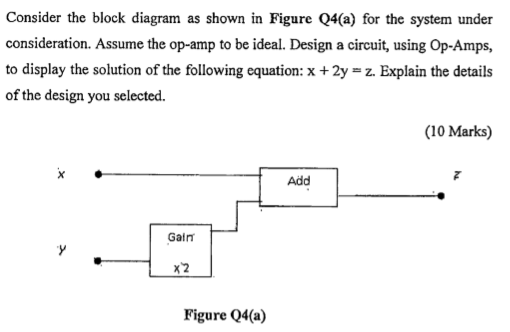Consider the block diagram as shown in Figure Q4(a) for the system under consideration. Assume the op-amp to be ideal. Design a circuit, using Op-Amps, to display the solution of the following equation: x + 2y = z. Explain the details of the design you selected. (10 Marks) Add Gain x2 Figure Q4(a)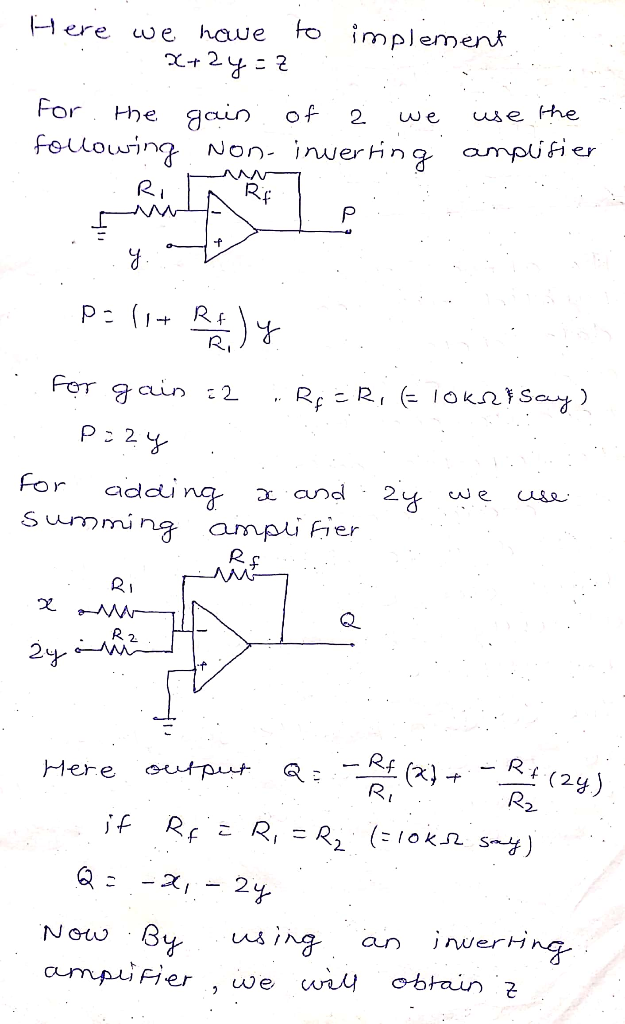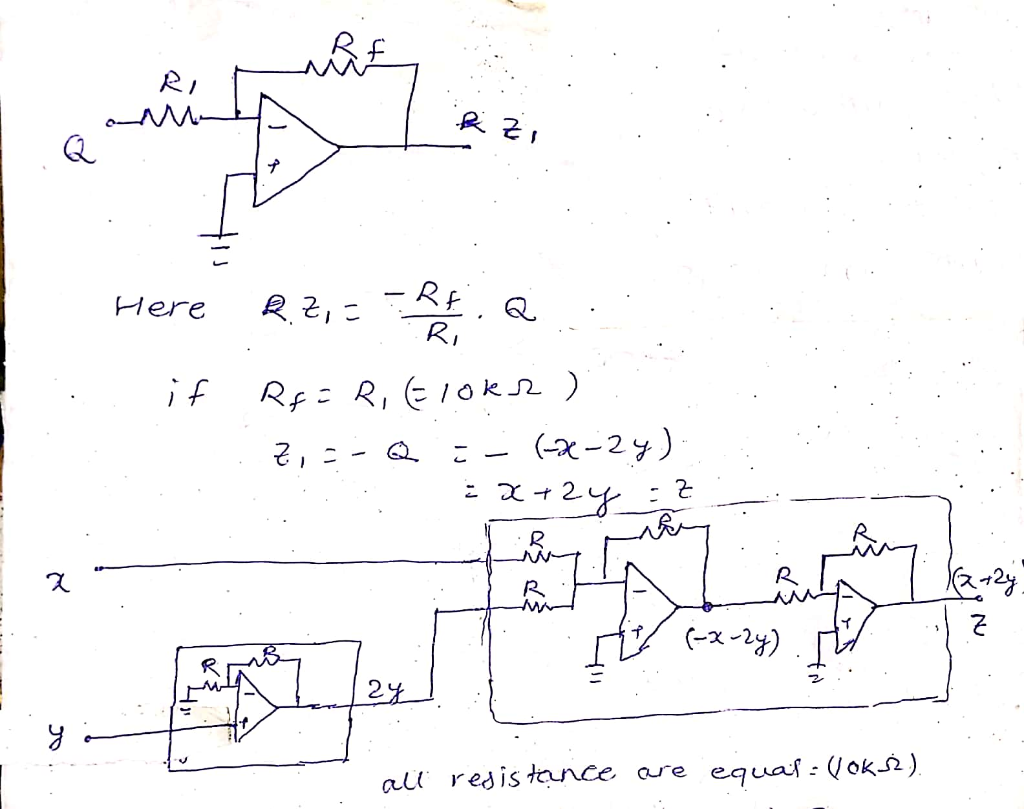#### Earn Coins

Coins can be redeemed for fabulous gifts.

Similar Homework Help Questions
• ### Question 4 (a) A feedback control system with a proportional controller is shown in Figure Q4...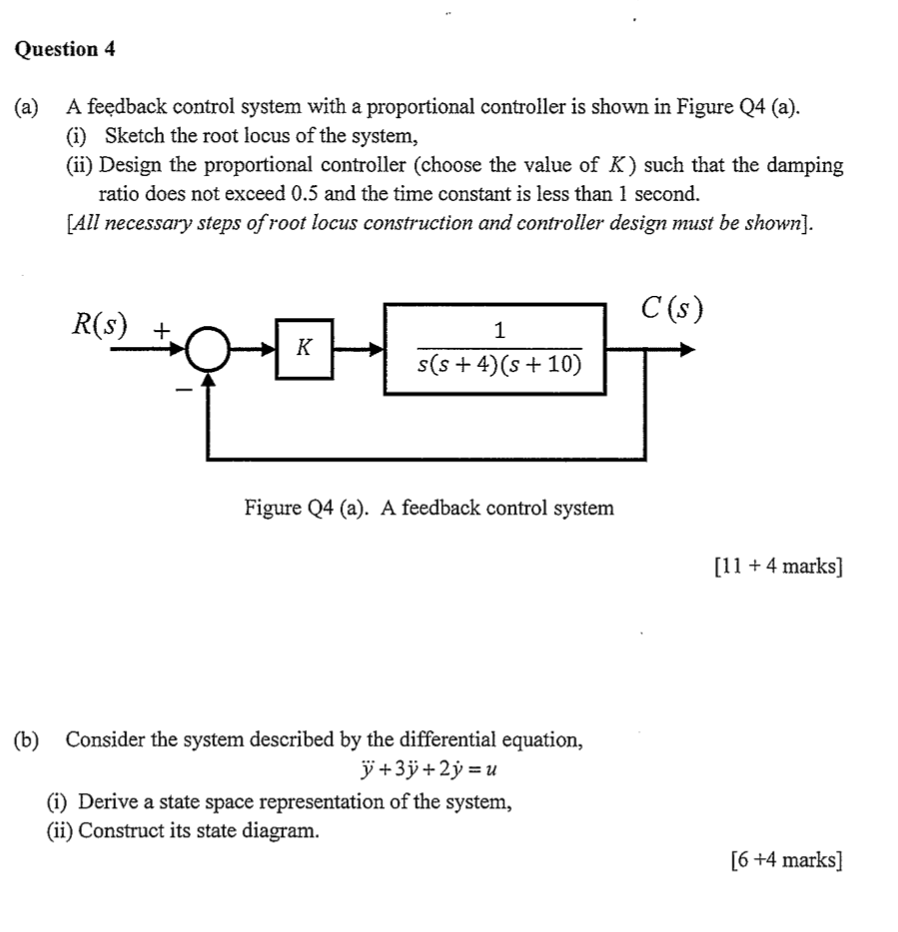Question 4 (a) A feedback control system with a proportional controller is shown in Figure Q4 (a). (i) Sketch the root locus of the system, (ii) Design the proportional controller (choose the value of K) such that the damping ratio does not exceed 0.5 and the time constant is less than 1 second. [All necessary steps of root locus construction and controller design must be shown). C(s) R(S) + s(s+4)(s + 10) Figure Q4 (a). A feedback control system [11...

• ### 2. Design a non-inverting op-amp circuit with two resistors under the following conditions: a. The gain of the ampl...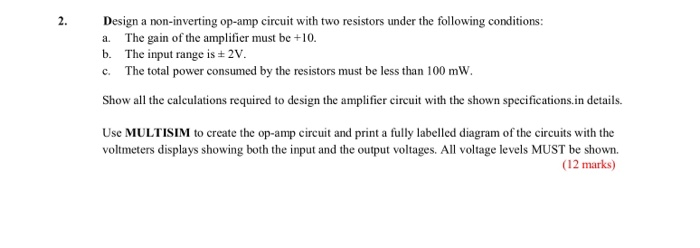2. Design a non-inverting op-amp circuit with two resistors under the following conditions: a. The gain of the amplifier must be +10 b. The input range is ± 2V c. The total power consumed by the resistors must be less than 100 mW Show all the calculations required to design the amplifier circuit with the shown specifications.in details Use MULTISIM to create the op-amp circuit and print a fully labelled diagram of the circuits with the voltmeters displays showing both...

• ### need help with C and D please The differentiator circuit shown in Figure 1 uses an op-amp with ideal characteristics. R2 R1 C1 Vi O Figure 1 (c) Sketch the Bode magnitude response for this circ...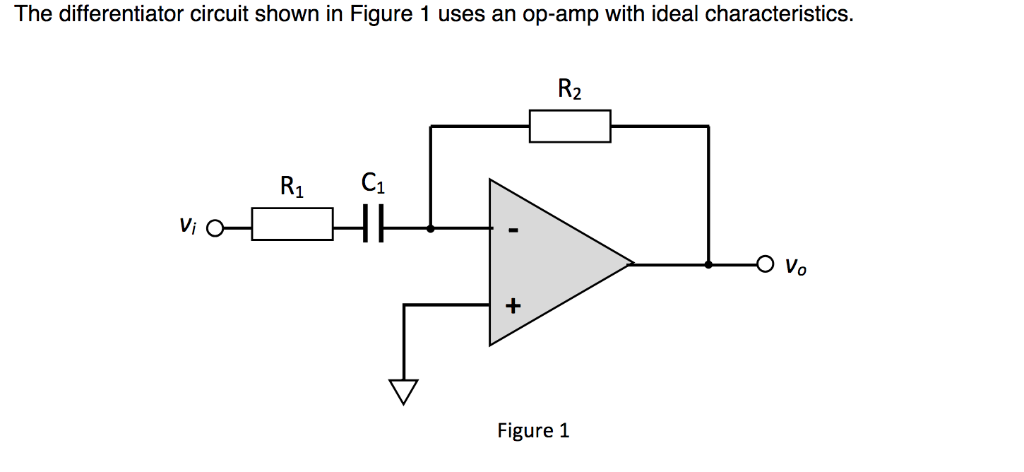need help with C and D please The differentiator circuit shown in Figure 1 uses an op-amp with ideal characteristics. R2 R1 C1 Vi O Figure 1 (c) Sketch the Bode magnitude response for this circuit for the frequency range of 10° to 108 Hz. (7 marks) (d) Sketch the output waveform of the differentiator and justify your answer, if v is as shown in Figure 2 with a period of: () 100 ms (ii) 10 us (6 marks) 0.11...

• ### ONLY NEED HELP WITH C AND D PLEASE! The differentiator circuit shown in Figure 1 uses an op-amp with ideal characteristics C1 Figure 1 (a) Prove that the gain of the circuit is given by the following...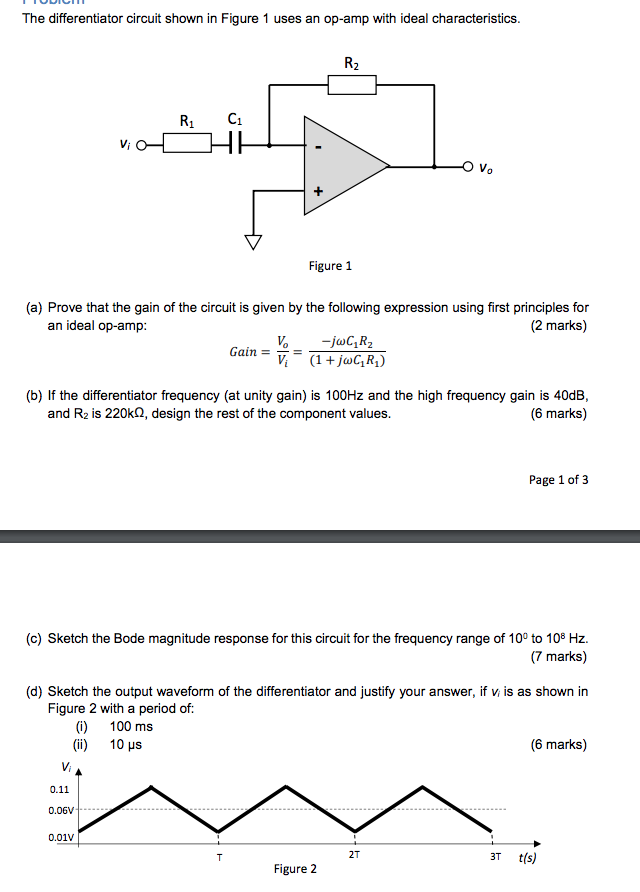ONLY NEED HELP WITH C AND D PLEASE! The differentiator circuit shown in Figure 1 uses an op-amp with ideal characteristics C1 Figure 1 (a) Prove that the gain of the circuit is given by the following expression using first principles for an ideal op-amp (2 marks) Gain = - (1 + juli R 1) (b) If the differentiator frequency (at unity gain) is 100Hz and the high frequency gain is 40dB and R2 is 220kQ, design the rest of...

• ### 4 (a) Based on the block diagram for a control system (Figure Q4(a) below) determine the...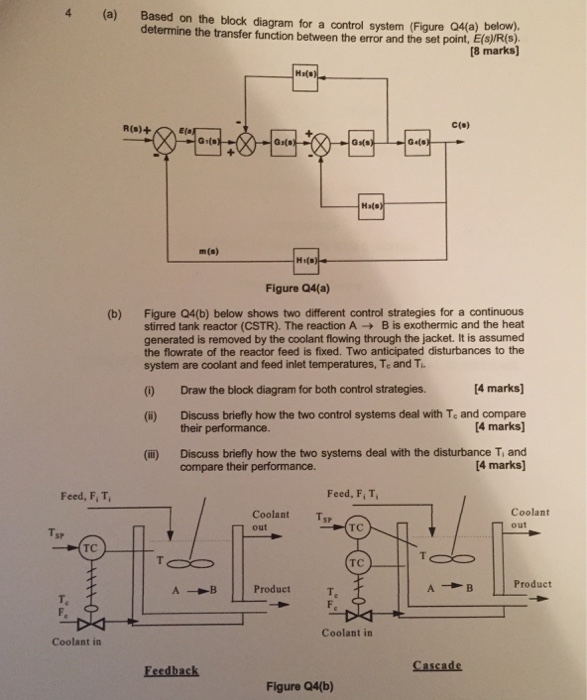4 (a) Based on the block diagram for a control system (Figure Q4(a) below) determine the transfer function between the error and the set point, E(s)/R(s) (8 marks) R(s)+ c(p) m(s) Figure Q4(a) Figure Q4(b) below shows two different control strategies for a continuous stirred tank reactor (CSTR). The reaction A → B is exothermic and the heat generated is removed by the coolant flowing through the jacket. It is assumed the flowrate of the reactor feed is fixed. Two...

• ### Q4. (a) Reduce the block diagram shown in Figure Q4a to a single mathematical expression suitable for implementation in MATLAB. Each letter represents a transfer function in the s-domain. (...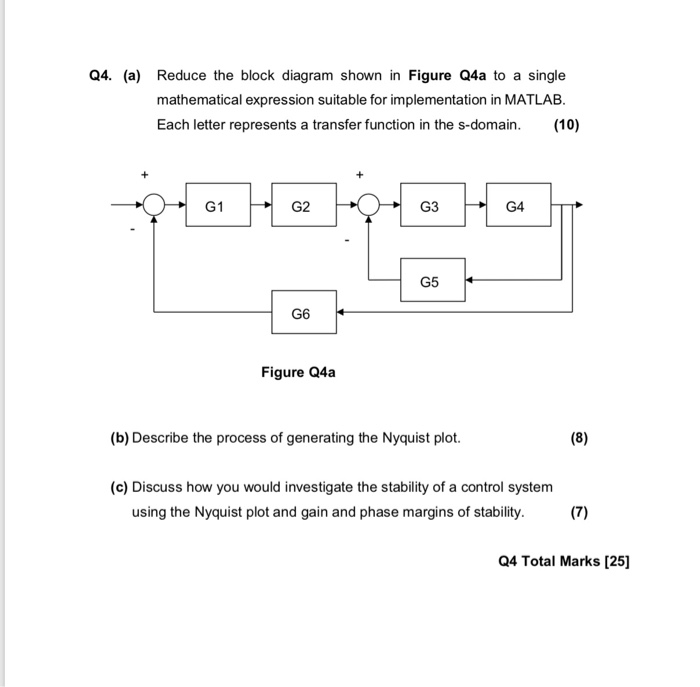Q4. (a) Reduce the block diagram shown in Figure Q4a to a single mathematical expression suitable for implementation in MATLAB. Each letter represents a transfer function in the s-domain. (10) G1 G2 G3 G4 G5 G6 Figure Q4a (b) Describe the process of generating the Nyquist plot. (c) Discuss how you would investigate the stability of a control system using the Nyquist plot and gain and phase margins of stability. (7) Q4 Total Marks  educe the block diagram shown...

• ### Problem 1) [15 marks] The gain of the dual-op-axap instrumentation amplifier shown in Fig. 1 can be adjusted by the variable resistor Ro. The op-amps are ideal. atu Fig. 1 a)Show that v.-2(1 RG )...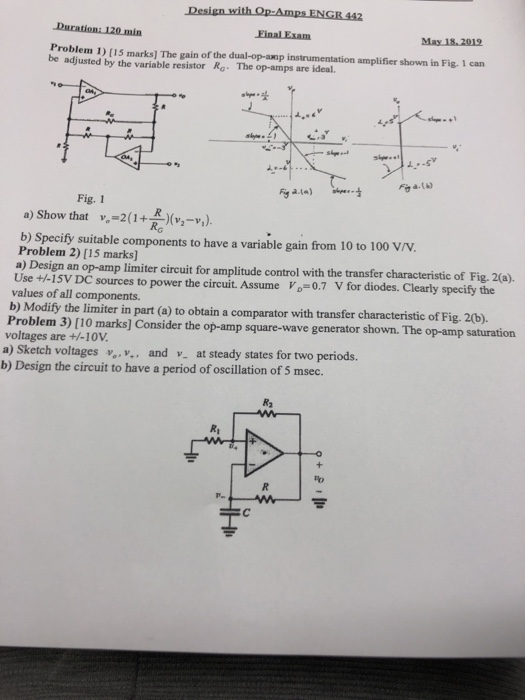Problem 1) [15 marks] The gain of the dual-op-axap instrumentation amplifier shown in Fig. 1 can be adjusted by the variable resistor Ro. The op-amps are ideal. atu Fig. 1 a)Show that v.-2(1 RG )(v2-v.). b Specify suitable components to have a variable gain from 10 to 100 V/V. Problem 2) [15 marks] a) Design an op-amp limiter circuit for amplitude control with the transfer characteristic of Fig. 2(a). Use +-15V DC sources to power the circuit. Assume Vo-0.7 V...

• ### Q4. (a) Reduce the block diagram shown in Figure Q4a to a single mathematical expression suitable...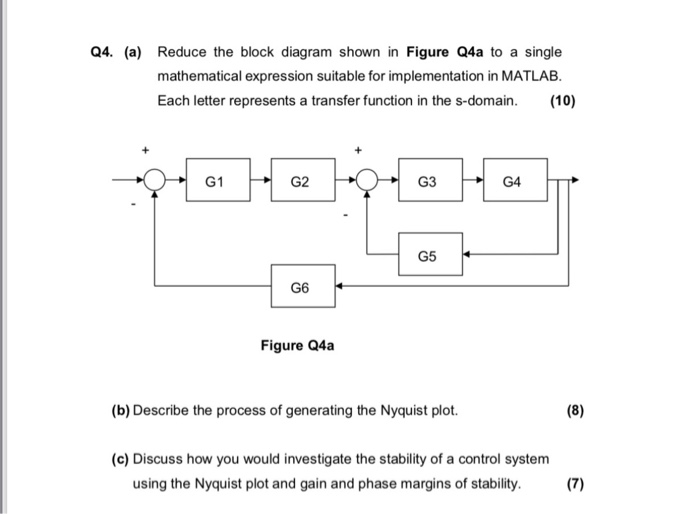Q4. (a) Reduce the block diagram shown in Figure Q4a to a single mathematical expression suitable for implementation in MATLAB. Each letter represents a transfer function in the s-domain. 10) G1G2 G3 G4 G5 G6 Figure Q4a (b) Describe the process of generating the Nyquist plot. (c) Discuss how you would investigate the stability of a control system using the Nyquist plot and gain and phase margins of stability. 7)

• ### having a de is to cascade Op amps wi Write an Figure P2.42 243. The objective...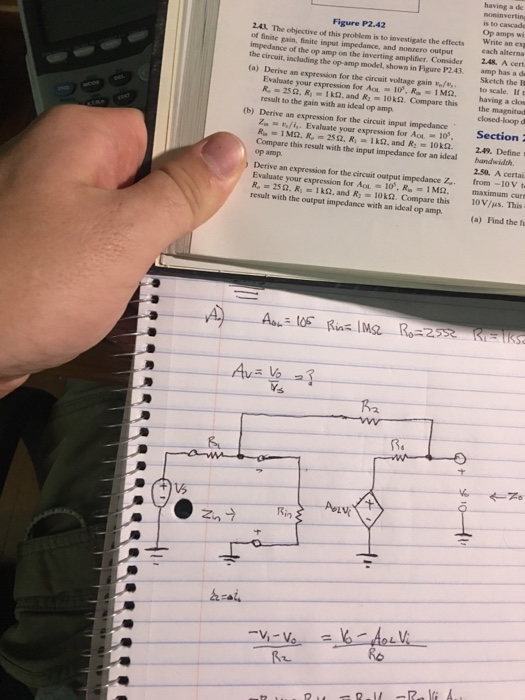having a de is to cascade Op amps wi Write an Figure P2.42 243. The objective of this probiem is to investigate the effects of finite gain, finite input impedance, and nonzero output impedance of the op amp on the inverting amplifier. Consider the effects ex 248. A cert the circui, including the op-amp model, shown in Figure P2.43. amp has a d (a) Derive an expression for the circuit voltage gain o/to scale. If t Sketch the E Evaluate...

• ### 5. A block diagram of a control system is shown in Figure 3. [10 marks] (a)...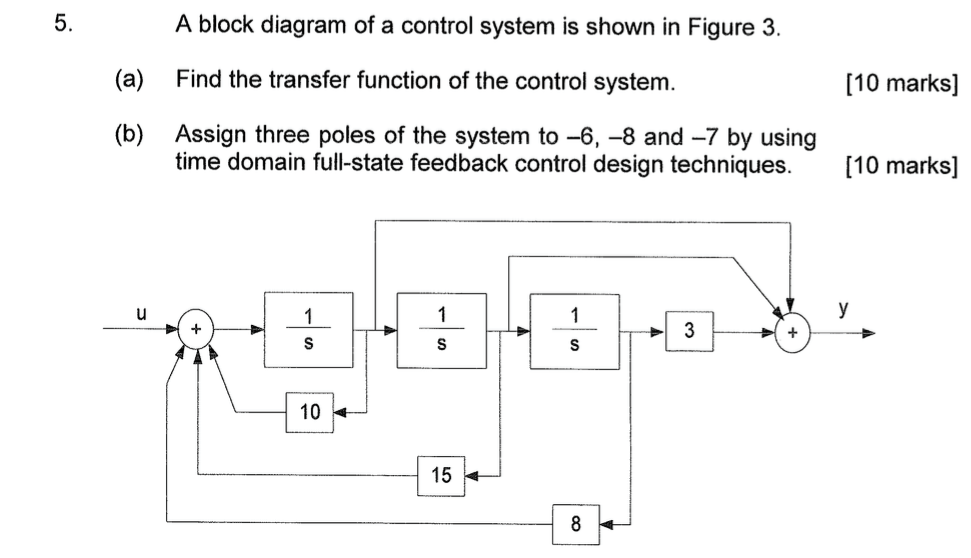5. A block diagram of a control system is shown in Figure 3. [10 marks] (a) Find the transfer function of the control system. (b) Assign three poles of the system to -6, -8 and -7 by using time domain full-state feedback control design techniques. 10 marks] 10 15